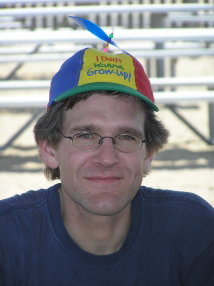Teaching

Thermodynamics and Statistical Physics

• Exam problems: pdf ps
• Exercises: pdf ps (diky F. Hinterleitnerovi)
• Old exam: pdf
• Old exam II: pdf

Statistical Physics and Thermodynamics

• Exam problems: pdf ps

Introduction to General Relativity

• Exam problems: pdf

Quantum Electrodynamics

• Feynman rules: pdf ps
• Assignment 1: pdf ps
• Assignment 2: pdf ps
• Lecture notes: pdf ps
• Exam problems: pdf ps